# Parallel Parking Using RRT Planner and MPC Tracking Controller

This example shows how to parallel park an ego car by generating a path using the RRT star planner and tracking the trajectory using nonlinear model predictive control (NLMPC).

### Parking Environment

In this example, the parking environment contains an ego vehicle and six static obstacles. The obstacles include four parked vehicles, the road curbside, and a yellow line on the road. The goal of the ego vehicle is to park at a target pose without colliding with any of the obstacles. The reference point for the ego vehicle pose is located at the center of rear axle.

The ego vehicle has two axles and four wheels. Define the ego vehicle parameters.

```vdims = vehicleDimensions; egoWheelbase = vdims.Wheelbase; distToCenter = 0.5*egoWheelbase;```

The ego vehicle starts at the following initial pose.

• X position of `7` m

• Y position of `3.1` m

• Yaw angle `0` rad

`egoInitialPose = [7,3.1,0];`

To park the center of the ego vehicle at the target location (X = `0`, Y = `0`) use the following target pose, which specifies the location of the rear-axle reference point.

• X position equal to half the wheelbase length

• Y position of `0` m

• Yaw angle `0` rad

`egoTargetPose = [-distToCenter,0,0];`

Visualize the parking environment. Specify a visualizer sample time of `0.1` s.

```Tv = 0.1; helperSLVisualizeParking(egoInitialPose,0);```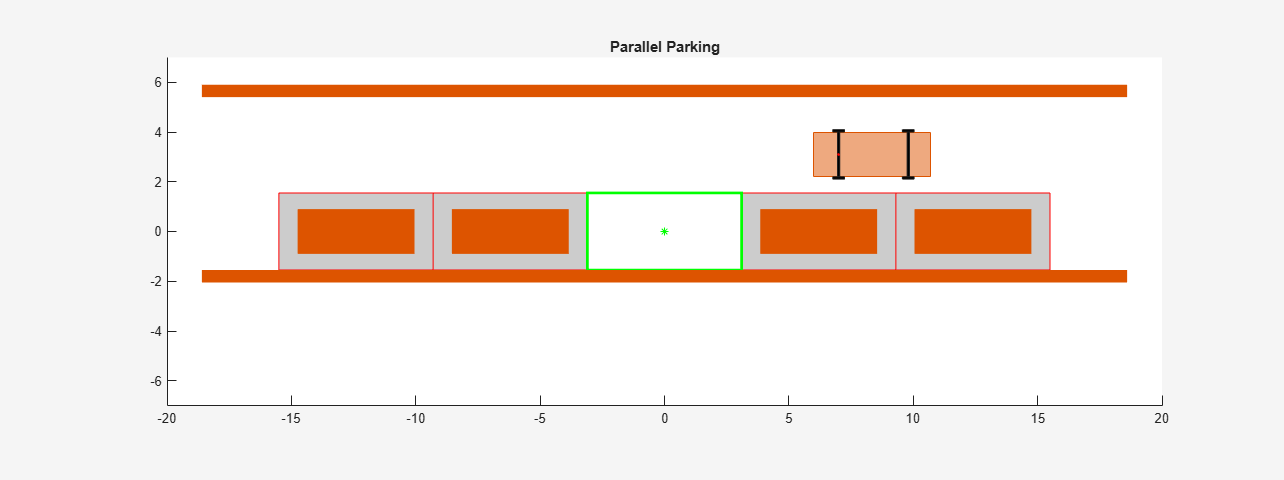In the visualization, the four parked vehicles are the orange boxes in the middle. The bottom orange boundary is the road curbside and the top orange boundary is the yellow line on the road.

### Ego Vehicle Model

For parking problems, the vehicle travels at low speeds. This example uses a kinematic bicycle model with front steering angle for the vehicle parking problem. The motion of the ego vehicle can be described using the following equations.

`$\begin{array}{l}\underset{}{\overset{˙}{x}}=v\cdot \mathrm{cos}\left(\psi \right)\\ \underset{}{\overset{˙}{y}}=v\cdot \mathrm{sin}\left(\psi \right)\\ \underset{}{\overset{˙}{\psi }}=\frac{v}{b}\cdot \mathrm{tan}\left(\delta \right)\end{array}$`

Here, $\left(\mathit{x},\mathit{y}\right)$ denotes the position of the vehicle and $\psi$ denotes the yaw angle of the vehicle. The parameter $b$ represents the wheelbase of the vehicle. $\left(x,y,\psi \right)$ are the state variables for the vehicle state functions. The speed $\mathit{v}$ and steering angle $\delta$ are the control variables for the vehicle state functions. The vehicle state functions are implemented in `parkingVehicleStateFcnRRT`.

### Path Planning from RRT Star

Configure the state space for the planner. In this example, the state of the ego vehicle is a three-element vector, [x y theta], with the $xy$ coordinates in meters and angle of rotation in radians.

```xlim = [-10 10]; ylim = [-2 6]; yawlim = [-3.1416 3.1416]; bounds = [xlim;ylim;yawlim]; stateSpace = stateSpaceReedsShepp(bounds); stateSpace.MinTurningRadius = 7;```

Create a custom state validator. The planner requires a customized state validator to enable collision checking between the ego vehicle and obstacles.

`stateValidator = parkingStateValidator(stateSpace);`

Configure the path planner. Use `plannerRRTStar` as the planner and specify the state space and state validator. Specify additional parameters for the planner.

```planner = plannerRRTStar(stateSpace,stateValidator); planner.MaxConnectionDistance = 4; planner.ContinueAfterGoalReached = true; planner.MaxIterations = 2000;```

Plan a path from the initial pose to the target pose using the configured path planner. Set the random number seed for repeatability.

```rng(9, 'twister'); [pathObj,solnInfo] = plan(planner,egoInitialPose,egoTargetPose);```

Plot the tree expansion on the parking environment.

```f = findobj('Name','Automated Parallel Parking'); ax = gca(f); hold(ax, 'on'); plot(ax,solnInfo.TreeData(:,1),solnInfo.TreeData(:,2),'y.-'); % tree expansion```

Generate a trajectory from `pathObj` by interpolating with an appropriate number of points.

```p = 100; pathObj.interpolate(p+1); xRef = pathObj.States;```

Draw the path on the environment.

`plot(ax,xRef(:,1), xRef(:,2),'b-','LineWidth',2)`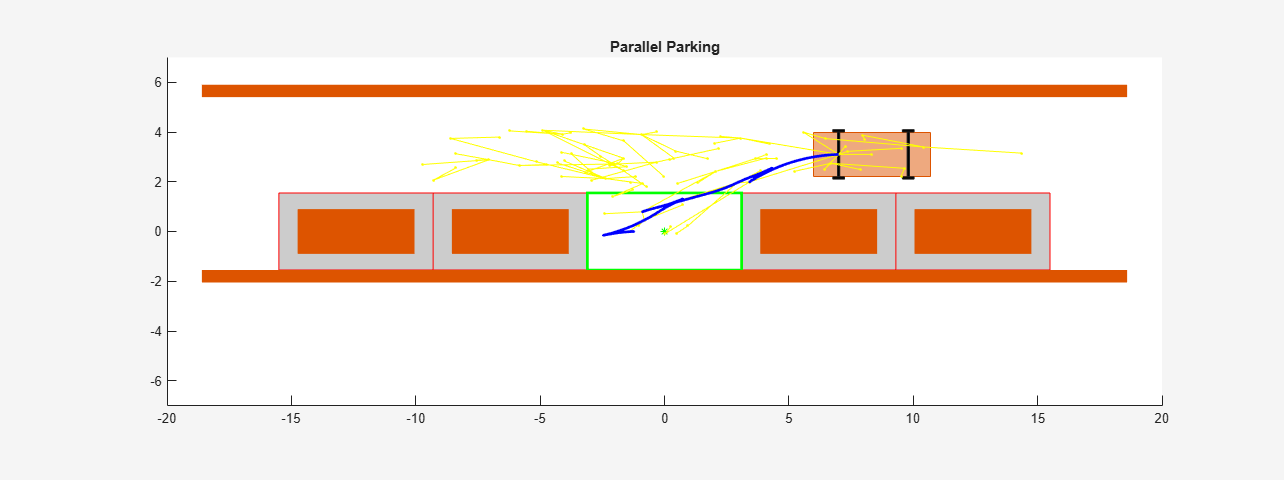### Design Nonlinear MPC Tracking Controller

Create the nonlinear MPC controller. For clarity, first disable the MPC command-window messages.

`mpcverbosity('off');`

Create the `nlmpc` controller object with three states, three outputs, and two inputs.

`nlobjTracking = nlmpc(3,3,2);`

Specify the sample time (`Ts`), prediction horizon (`PredictionHorizon`), and control horizon (`ControlHorizon`) for the controller.

```Ts = 0.1; pTracking = 10; nlobjTracking.Ts = Ts; nlobjTracking.PredictionHorizon = pTracking; nlobjTracking.ControlHorizon = pTracking;```

Define constraints for the manipulated variables. Here, `MV(1)` is the ego vehicle speed in m/s, and `MV(2)` is the steering angle in radians.

```nlobjTracking.MV(1).Min = -2; nlobjTracking.MV(1).Max = 2; nlobjTracking.MV(2).Min = -pi/6; nlobjTracking.MV(2).Max = pi/6;```

Specify tuning weights for the controller.

```nlobjTracking.Weights.OutputVariables = [1,1,3]; nlobjTracking.Weights.ManipulatedVariablesRate = [0.1,0.2];```

The motion of ego vehicle is governed by a kinematic bicycle model. Specify the controller state function and the state-function Jacobian.

```nlobjTracking.Model.StateFcn = "parkingVehicleStateFcnRRT"; nlobjTracking.Jacobian.StateFcn = "parkingVehicleStateJacobianFcnRRT";```

Specify terminal constraints on control inputs. Both speed and steering angle are expected to be zero at the end.

`nlobjTracking.Optimization.CustomEqConFcn = "parkingTerminalConFcn";`

Validate the controller design.

`validateFcns(nlobjTracking,randn(3,1),randn(2,1));`
```Model.StateFcn is OK. Jacobian.StateFcn is OK. No output function specified. Assuming "y = x" in the prediction model. Optimization.CustomEqConFcn is OK. Analysis of user-provided model, cost, and constraint functions complete. ```

### Run Closed-loop Simulation in MATLAB

To speed up simulation, first generate a MEX function for the NLMPC controller.

Specify the initial ego vehicle state.

`x = egoInitialPose';`

Specify the initial control inputs.

`u = [0;0];`

Obtain code generation data for the NLMPC controller.

`[coredata,onlinedata] = getCodeGenerationData(nlobjTracking,x,u);`

Build a MEX function for simulating the controller.

`mexfcn = buildMEX(nlobjTracking,'parkingRRTMex',coredata,onlinedata);`
```Generating MEX function "parkingRRTMex" from nonlinear MPC to speed up simulation. Code generation successful. MEX function "parkingRRTMex" successfully generated. ```

Initialize data before running simulation.

```xTrackHistory = x; uTrackHistory = u; mv = u; Duration = 14; Tsteps = Duration/Ts; Xref = [xRef(2:p+1,:);repmat(xRef(end,:),Tsteps-p,1)];```

Run the closed-loop simulation in MATLAB using the MEX function.

```for ct = 1:Tsteps % States xk = x; % Compute optimal control moves with MEX function onlinedata.ref = Xref(ct:min(ct+pTracking-1,Tsteps),:); [mv,onlinedata,info] = mexfcn(xk,mv,onlinedata); % Implement first optimal control move and update plant states. ODEFUN = @(t,xk) parkingVehicleStateFcnRRT(xk,mv); [TOUT,YOUT] = ode45(ODEFUN,[0 Ts], xk); x = YOUT(end,:)'; % Save plant states for display. xTrackHistory = [xTrackHistory x]; %#ok<*AGROW> uTrackHistory = [uTrackHistory mv]; end```

Plot and animate the simulation results when using the NLMPC controller. The tracking results match the reference trajectory from the path planner.

`plotAndAnimateParkingRRT(p,xRef,xTrackHistory,uTrackHistory);`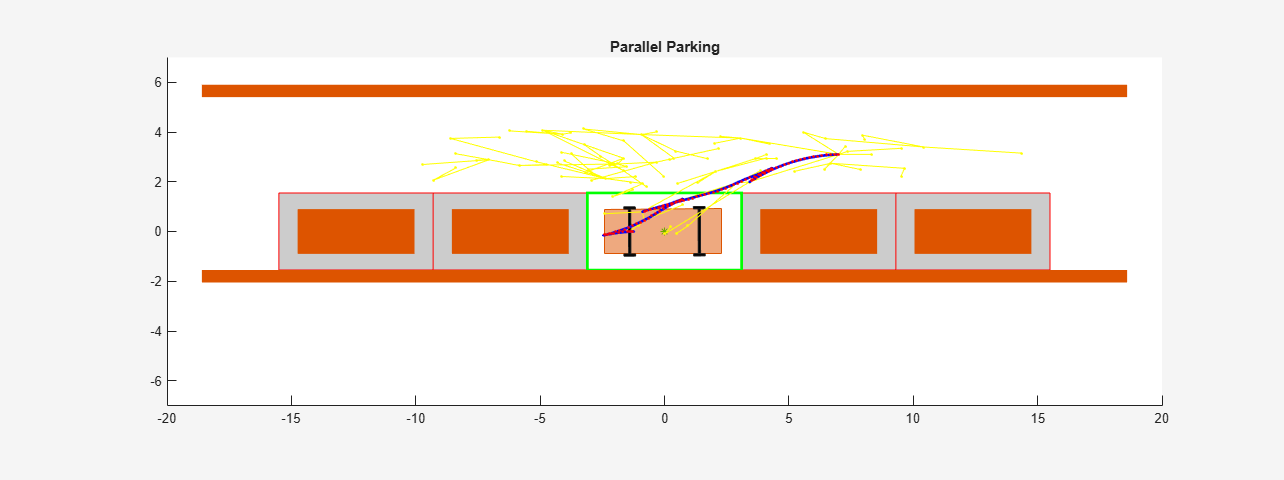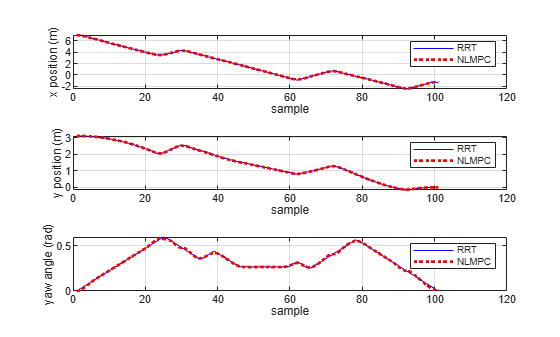```Tracking error infinity norm in x (m), y (m) and theta (deg): 0.0538, 0.0538, 1.3432 Final control inputs of speed (m/s) and steering angle (deg): 0.0001, 0.0981 ```

### Run Closed-loop Simulation in Simulink

To simulate the NLMPC controller in Simulink ®, use the Nonlinear MPC Controller block. For this example, to simulate the ego vehicle, use the Vehicle Body 3DOF Lateral block, which is a Bicycle Model (Automated Driving Toolbox) block.

```mdl = 'mpcVDAutoParkingRRT'; open_system(mdl)```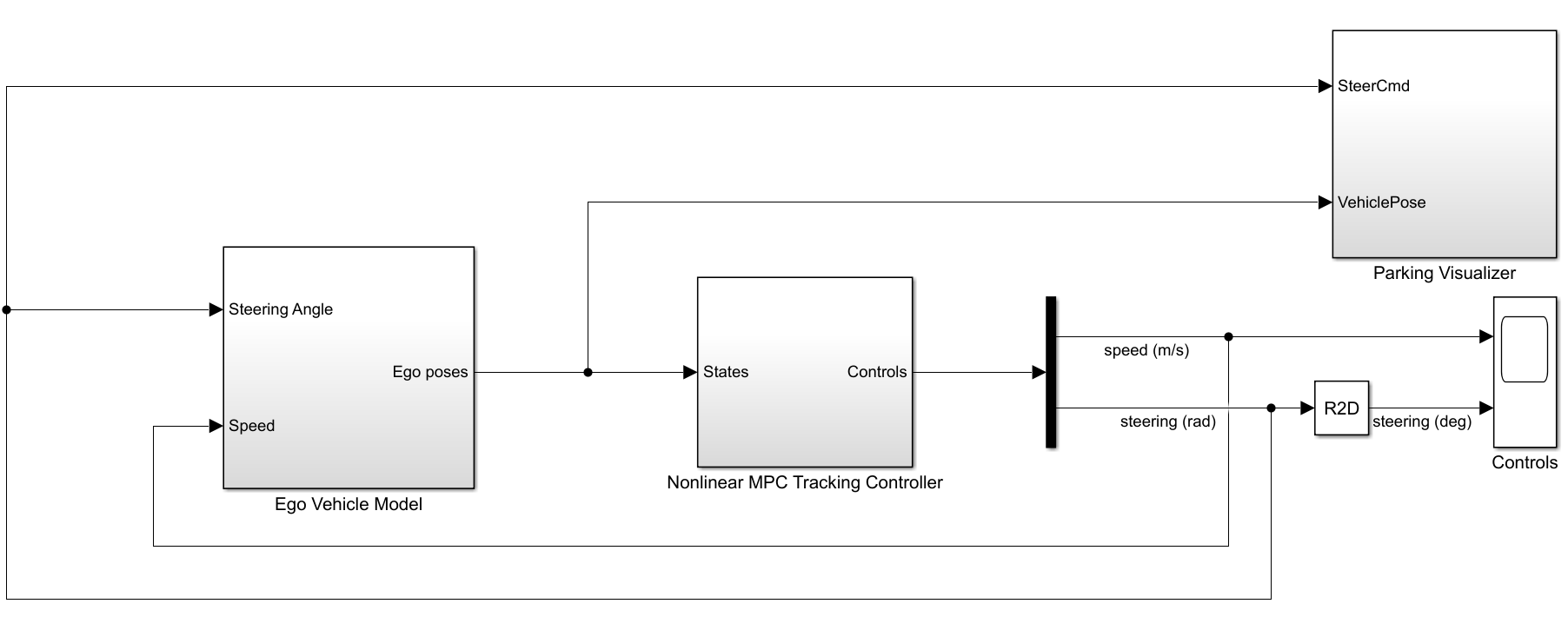Close the animation plot before simulating the model.

```f = findobj('Name','Automated Parallel Parking'); close(f)```

Simulate the model.

`sim(mdl)`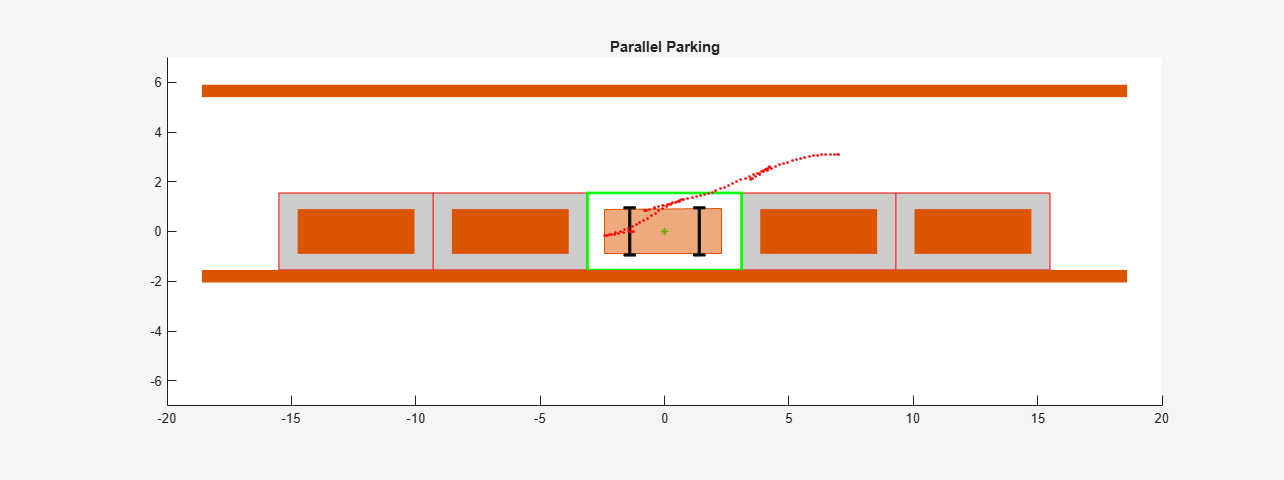```ans = Simulink.SimulationOutput: tout: [7659x1 double] SimulationMetadata: [1x1 Simulink.SimulationMetadata] ErrorMessage: [0x0 char] ```

Examine the Ego Vehicle Pose and Controls scopes. The simulation results are similar to the MATLAB simulation. The ego vehicle has parked at the target pose successfully without collisions with any obstacles.

### Conclusion

This example shows how to how to parallel park an ego car by generating a path using an RRT star planner and tracking the trajectory using a nonlinear MPC controller. The controller navigates the ego vehicle to the target parking spot without colliding with any obstacles.

```% Enable message display mpcverbosity('on'); % Close Simulink model bdclose(mdl) % Close animation plots f = findobj('Name','Automated Parallel Parking'); close(f)```